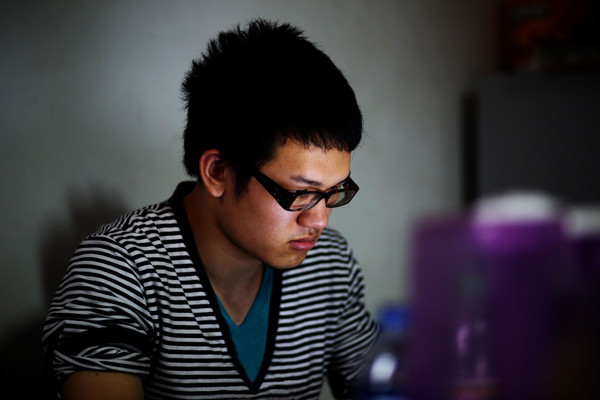IEEE多媒体与博览会国际会议（ICME）每年有大约1,000名作者和500名参与者参加，这是由四个IEEE协会主办的联盟多媒体会议。它是一个促进交流多媒体最新进展的论坛从电路和系统，通信，计算机和信号处理社区的研究和开发角度来看的技术，系统和应用程序。 官网地址：http://dblp.uni-trier.de/db/conf/icmcs/

热门内容0520

最新内容000

最新论文Uncertainty involved in computational materials modeling needs to be quantified to enhance the credibility of predictions. Tracking the propagation of model-form and parameter uncertainty for each simulation step, however, is computationally expensive. In this paper, a multiscale stochastic reduced-order model (ROM) is proposed to propagate the uncertainty as a stochastic process with Gaussian noise. The quantity of interest (QoI) is modeled by a non-linear Langevin equation, where its associated probability density function is propagated using Fokker-Planck equation. The drift and diffusion coefficients of the Fokker-Planck equation are trained and tested from the time-series dataset obtained from direct numerical simulations. Considering microstructure descriptors in the microstructure evolution as QoIs, we demonstrate our proposed methodology in three integrated computational materials engineering (ICME) models: kinetic Monte Carlo, phase field, and molecular dynamics simulations. It is demonstrated that once calibrated correctly using the available time-series datasets from these ICME models, the proposed ROM is capable of propagating the microstructure descriptors dynamically, and the results agree well with the ICME models.000下载预览
Top微信扫码咨询专知VIP会员